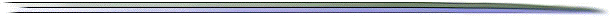# The Cost Constraint as a Cost Driver

from the Perspective of Competitive Advantage

by
Edwin B. DeanFor many years, in the parametric cost analysis community, the word cost driver has been used to denote a measure which is statistically significant in an equation which relates cost to a set of noncost measures. System weight, which is a measure of size, for example, is usually statistically significant for a hardware system. It was not until I began to address the minimization of cost that a second type of cost driver arose within a new and very important context, the existence of cost forces which arise from constraints.

The constrained cost minimization problem may be stated as

minimize f(x)

over x

subject to g(x) >= a

where f(x) is life cycle cost in terms of conventional cost drivers xi which are components of the vector x, g(x) is a vector of system constraints gi(x), a is a constant vector, and f(x) and gi(x) are to be viewed as generalized coordinates.

At the minimum the following equation is satisfied.

Dxf(x) = r * Dxg(x)

where Dx represents the partial derivative operator, Dxh(x) is the gradient of the function h(x), r is the Lagrange multiplier vector, and * is the vector dot product.

This is Newtons third law for cost where ri Dxgi(x) is the ith reactive cost force with magnitude ri |Dxgi(x)| and direction Dxgi(x) / |Dxgi(x)|. Dxf(x) is the driving cost force with magnitude |Dxf(x)| and direction Dxf(x) / |Dxf(x)|. It is the gradient of the potential f(x) which is the life cycle cost. Note that ri is nonzero at equilibrium (the minimum) only if gi(x) = ai. If gi(x) = ai, then gi(x) is the generalized coordinate of the reactive cost force (driver) ri Dxgi(x). To reduce the life cycle cost f(x), gi(x)-ai must be relaxed.

The cost force concept has been derived from many places, including personal experience in analogic thought as an analog computer programmer, but, in particular, from the very excellent description of mechanics by Lanczos (1949) and the very excellent description of generalized coordinates by Beyerly (1916).#### References

• Beyerly, W. E. (1916). An Introduction to the Use of Generalized Coordinates in Mechanics and Physics, Ginn and Company, New York NY, republished in 1965 by Dover Publications, New York NY.
• Lanczos, C. (1949). The Variational Principles of Mechanics, University of Toronto Press, Toronto, Canada.